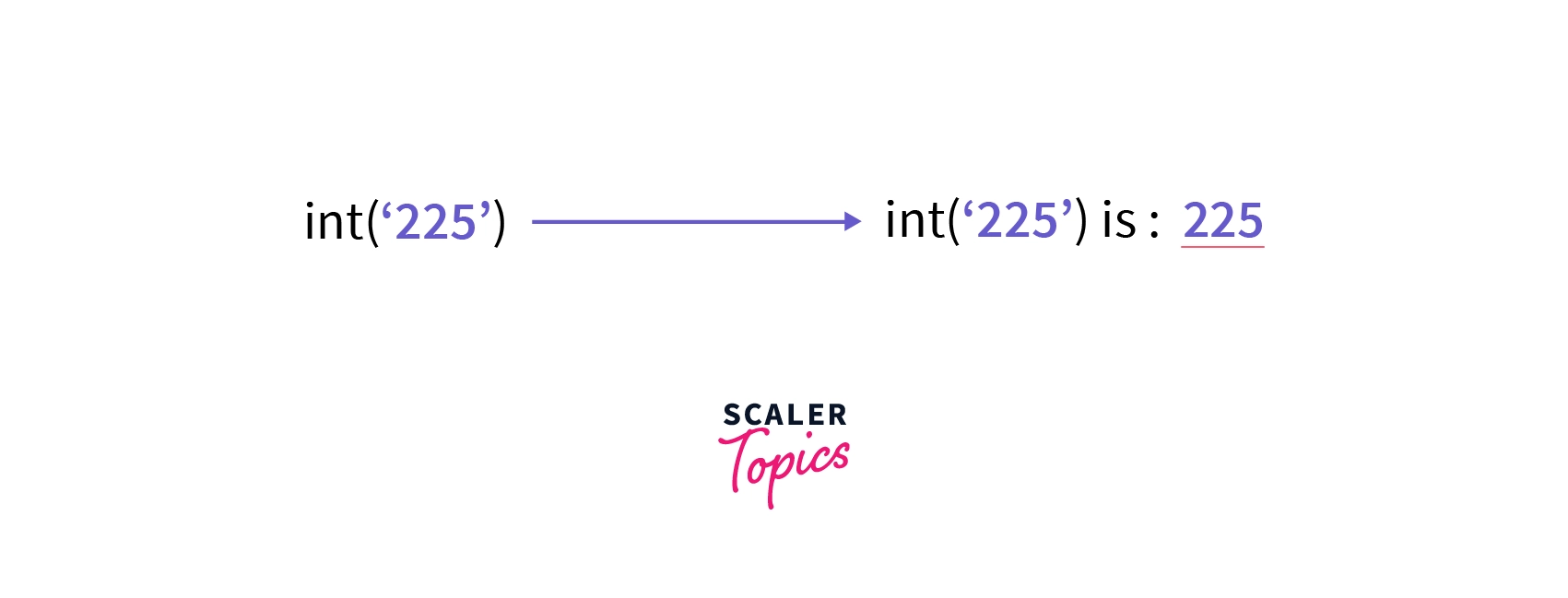# String to int in Python

Challenge Inside! : Find out where you stand! Try quiz, solve problems & win rewards!
Learn via video coursePython Course for Beginners With Certification: Mastering the Essentials
By Rahul JanghuFree
4.90Enrolled: 1000Python Course for Beginners With Certification: Mastering the Essentials
Rahul JanghuFree
4.90
Enrolled: 1000

## Overview

The conversion of one data type into the other data type is known as type casting or type conversion. To convert string to int, we use the int() method in python. To convert a string into a floating-point number, we use the float() method in python.

## How to Convert String to int in Python?

The conversion of one data type into the other data type is known as type casting or type conversion. To convert string to int, we use the int() method in python. To convert a string into a floating-point number, we use the float() method in python.

The int in python is a built-in method that convert string to int. The int() method can convert a string or a number into an integer. We can also use int in python to convert binary numbers to decimal numbers, hexadecimal numbers to decimal numbers, and octal numbers into decimal numbers.

The int() method raises an error when a non-string or non-integer data type is given as a parameter. The built-in raised error is termed as TypeError.

Similarly, we can use the float() method to convert an integer or specific strings into floating-point numbers. The float() method takes string or integer data type and converts the given data into a floating-point number. If a string is passed which is not a decimal point number or strings mentioned above, then ValueError is raised.

## Using int() Function

We can convert string to int using the Python's built-in method called the int() method. The int() method takes a string or integer data type and converts the given data into an integer number.

The syntax of the method, int in python is very simple:The value parameter is required but the base parameter is optional.

The int in python converts string to int and returns the integer object value of the given string or floating number. If we do not give any parameter in the method, it will return 0.

The exception raised by int in python is known as TypeError.

Whenever we pass a data type other than a string or an integer, the int in python raises an error.

For example, if we try to the typecast list using int in python.

Example:

Let us convert a floating-point number into an integer using the method, int in python.

Output:

## Using float() Function

We can convert an integer or specific strings into floating-point numbers using the python built-in method called float() method. The float() method takes string or integer data type and converts the given data into a floating-point number.

The syntax of the method, int in python is very simple:

The value parameter is optional.

Example:

Let us convert an integer number into a floating-point number using the method, float in python.

Output: